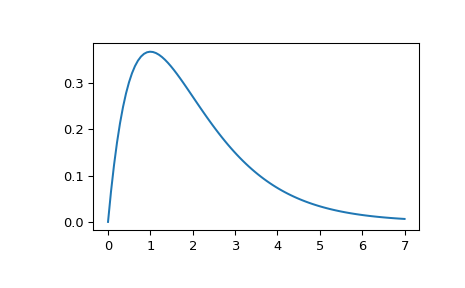# scipy.signal.impulse¶

scipy.signal.impulse(system, X0=None, T=None, N=None)[source]

Impulse response of continuous-time system.

Parameters
systeman instance of the LTI class or a tuple of array_like

describing the system. The following gives the number of elements in the tuple and the interpretation:

• 1 (instance of lti)

• 2 (num, den)

• 3 (zeros, poles, gain)

• 4 (A, B, C, D)

X0array_like, optional

Initial state-vector. Defaults to zero.

Tarray_like, optional

Time points. Computed if not given.

Nint, optional

The number of time points to compute (if T is not given).

Returns
Tndarray

A 1-D array of time points.

youtndarray

A 1-D array containing the impulse response of the system (except for singularities at zero).

Notes

If (num, den) is passed in for system, coefficients for both the numerator and denominator should be specified in descending exponent order (e.g. s^2 + 3s + 5 would be represented as [1, 3, 5]).

Examples

Second order system with a repeated root: x’‘(t) + 2*x(t) + x(t) = u(t)

>>> from scipy import signal
>>> system = ([1.0], [1.0, 2.0, 1.0])
>>> t, y = signal.impulse2(system)
>>> import matplotlib.pyplot as plt
>>> plt.plot(t, y)#### Previous topic

scipy.signal.lsim2

#### Next topic

scipy.signal.impulse2